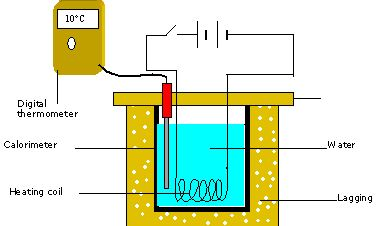Physics

# Descreibe Joule’s Law of Heating Effect

In a conductor, the free electrons are always at random motion making collisions with ions or atoms of the conductor. When a voltage V is applied between the ends of the conductor, resulting in the flow of current I, the free electrons are accelerated. Hence the electrons gain energy at the rate of VI per second. The lattice ions or atoms receive this energy VI from the colliding electrons in random bursts.This increase in energy is nothing but the thermal energy of the lattice. Thus for a steady current I, the amount of heat produced in time t is

H = VIt … … (1)

For a resistance R,

H = I2Rt … … (2) and

H = (V2/R) . t … … (3)

The above relations were experimentally verified by Joule and are known as Joule’s law of heating. By equation (2) Joule’s law implies that the heat produced is (i) directly proportional to the square of the current for a given R (ii) directly proportional to resistance R for a given I and (iii) directly proportional to the time of passage of current. Also by equation (3), the heat produced is inversely proportional to resistance R for a given V.